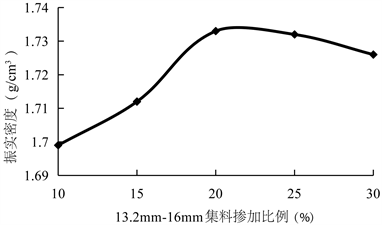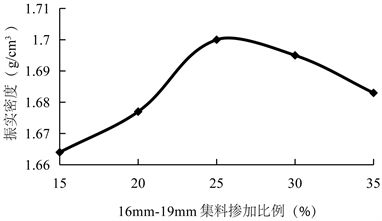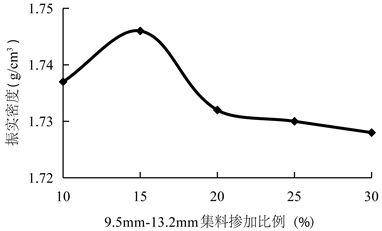﻿ 基于连通孔隙率与强度的多孔混凝土配合比优化设计方法研究

# 基于连通孔隙率与强度的多孔混凝土配合比优化设计方法研究Research on Optimum Design Method of Mix Proportion of Porous Concrete Based on Connected Porosity and Strength

Abstract: As a kind of drainage-type base material, porous concrete can effectively exclude the water inside the road structure. In this paper, the connected porosity is used as the design index, and the 7 days compressive strength of concrete is used as the design goal. The design method of porous concrete’s mixture: grading design - target porosity determination - calculation of mix proportion - concrete strength test was proposed; the best forming method of the specimen was determined by orthogonal test; connected porosity, which is an important factor affecting drainage performance of porous concrete, was tested; and the relationship between the target porosity and the connected porosity was analyzed. The results of calculation and experiment show that the connected porosity of porous concrete can be designed by the volume of cement slurry, and the difference between the designed target porosity and the measured connected porosity is only 0.3%~2.6%. It is shown that the connected porosity of concrete can be designed by setting the target porosity and the final porous concrete mix proportion can be determined by the 7 days compressive strength of concrete.

1. 引言

2. 原材料

2.1. 粗集料

2.2. 水泥Table 1. The summary table of coarse aggregate performance test resultsTable 2. The summary table of cement chemical composition analysis results

3. 多孔混凝土配合比优化设计

3.1. 试件制备方法

3.2. 级配设计

3.2.1. 集料的堆积密度

3.2.2. 逐级填充

① 第一级填充

② 第二级填充

③ 第三级填充Table 3. The summary table of coarse aggregate density test resultsFigure 1. The result of first stage fillFigure 2. The result of second stage fillFigure 3. The result of third stage fill

3.3. 配合比计算Table 4. The table of porous concrete gradeTable 5. The table of porous concrete mixed aggregate characteristics

${m}_{g}=0.98\rho$ (1)

${V}_{c+w}={P}^{\prime }-P$ (2)

${m}_{c}/{\rho }_{c}+{m}_{w}/{\rho }_{w}={P}^{\prime }-P$ (3)

3.4. 水灰比、拌和时间、振动时间的确定

3.4.1. 试验影响因素、水平的确定

3.4.2. 正交试验表设计及正交试验结果Table 6. The table of orthogonal test factors and levelsTable 7. The table of orthogonal test design and orthogonal test result

3.4.3. 极差分析

Kj—第j列同一水平出现的次数，等于试验次数n (n = 16)/第j列水平数；

Ij/kj~IVj/kj—第j列各水平所对应的试验指标的平均值；

Dj—每个因素所对应的平均极差，第j列各水平所对应的平均值中的最大值与最小值的差。Table 8. The table of orthogonal test range analysis

3.5. 多孔混凝土试件成型参数

3.6. 多孔混凝土的抗压强度和抗弯拉强度

4. 多孔混凝土连通孔隙率与目标孔隙率的关系研究

4.1. 多孔混凝土连通孔隙率测试方法

${n}_{\text{e}}=\left(1-\frac{m{}_{2}-{m}_{1}}{V{\rho }_{w}}\right)×100$ (4)

m1—试件的水中重(g)；

m2—试件的空气中重(g)；

V—试件的体积(cm3)；Table 9. The summary table of porous concrete mix proportion design results (per cubic metre)Table 10. The summary table of porous concrete 7 d compressive strength and flexural strength results

ρw—水的密度，一般取1 g/cm3

4.2. 连通孔隙率与目标孔隙率关系分析

$y=kx+b$ (5)

x—即目标孔隙率(%)；

k—一次项系数；

b—常数项。Table 11. The table of specimen connectivity porosity test resultsTable 12. The table of linear fitting formula

5. 结论

1) 通过正交试验确定出多孔混凝土试件成型时水灰比、拌和时间、振动时间的最佳参数分别为0.26、40 s、30 s。

2) 实测的连通孔隙率与目标孔隙率两者之间差值仅为0.3%~2.6%，且存在很好的线性关系，线性拟合公式中k值的取值范围为0.9~1.1，b值的取值范围为0.5~2.5。

3) 根据振实后集料的密度，确定混合集料的间隙率，采用此间隙率与目标孔隙率的差值能够确定单位体积多孔混凝土中需要的水泥浆填充的体积，用于计算多孔混凝土的连通孔隙率。

 邓学钧. 路基路面工程(第三版) [M]. 北京: 人民交通出版社, 2008.

 Tarefder, R.A. and Ahmad, M. (2015) Evaluation of Pore Structure and Its Influence on Permeability and Moisture Damage in Asphalt Concrete. International Journal of Pavement En-gineering, 18, 274-283.

 Caestecker. (1997) Test Section of Noiseless Cement Pavement. Proceedings of 6th International Purdue Conference on Concrete Design and Material for High Performance, Indianapolis, 13.

 Fuchs, D.G. and Buys, F.R. (1993) Noise-Reducing Concrete Pavements. Proceedings of 5th International Conference on Concrete Design and Rehabilitation, Purdue University, 25-30.

 彭运朝. 多孔混凝土研究综述[J]. 农业科技与装备, 2012, 217(7): 64-65.

 舒翔, 陈少幸. AASHTO 2002高速公路路面结构层防排水系统设计[J]. 广东公路交通, 2009, 25(5): 17-19.

 郑木莲, 陈拴发, 王秉刚. 基于正交试验的多孔混凝土配合比设计方法[J]. 同济大学学报(自然科学版), 2006, 36(10): 1319-1323, 1339.

 郑木莲. 多孔混凝土排水基层研究[D]: [博士学位论文]. 西安: 长安大学, 2004.

 JTG E42-2005. 公路工程集料试验规程[S]. 北京: 人民交通出版社, 2005.

 JTG/T F20-2015. 公路路面基层施工技术细则[S]. 北京: 人民交通出版社, 2015.

Top